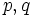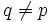# Centralizer product theorem

## Statement

### For faithful group actions

Suppose$p,q$ are distinct primes. Let$P$ be a finite$p$-group, and$Q \le \operatorname{Aut}(P)$ be an abelian$q$-group that is not cyclic. Suppose$x_1, x_2, \dots, x_n$ are the non-identity elements of$Q$, enumerated in any arbitrary order. Then, we have:$P = C_P(x_1)C_P(x_2) \dots C_P(x_n)$.

### For general group actions

Suppose$p,q$ are distinct primes. Let$P$ be a finite$p$-group, and$Q$ be an abelian$q$-group that is not cyclic. Suppose$Q$ acts on$P$ by automorphisms. Suppose$x_1, x_2, \dots, x_n$ are the non-identity elements of$Q$, enumerated in any arbitrary order. Then, we have:$P = C_P(x_1)C_P(x_2) \dots C_P(x_n)$.

Note that the version for general group actions follows directly from the version for faithful group actions. In fact, for an action that is not faithful, it suffices to take the products of centralizers of coset representatives of the kernel of the action.

## Proof

This proof uses a tabular format for presentation. Provide feedback on tabular proof formats in a survey (opens in new window/tab) | Learn more about tabular proof formats|View all pages on facts with proofs in tabular format
This proof uses the principle of mathematical induction in a nontrivial way (i.e., it would be hard to write the proof clearly without explicitly using induction).

Given: Primes$p \ne q$. A finite$p$-group$P$. An abelian non-cyclic subgroup$Q \le \operatorname{Aut}(P)$.$x_1, x_2, \dots, x_n$ are the non-identity elements of$Q$, enumerated in any order.

To prove:$P = C_P(x_1)C_P(x_2) \dots C_P(x_n)$.

Proof: We prove this by induction on the order of$P$, combined with fact (1). Fact (1) settles the case for$P$ an elementary abelian$p$-group.

Step no. Assertion/construction Facts used Given data used Previous steps used Explanation
1 Let$R = \Omega_1(Z(P))$; in other words,$R$ is the subgroup of$P$ comprising the identity element and elements of order$p$ in the center of$p$. Then,$Q$ acts on$R$ by automorphisms, and$R$ is a nontrivial elementary abelian$p$-group. Fact (2)$P$ is a finite$p$-group [SHOW MORE]
2 There exists a non-identity$g \in Q$ such that$C_R(g)$ is nontrivial Fact (1)$Q$ is a finite abelian non-cyclic$q$-group,$q \ne p$. Step (1) [SHOW MORE]
3 With$g$ selected as in Step (2),$C_R(g)$ is a normal subgroup of$P$ Fact (3) Steps (1), (2) [SHOW MORE]
4$Q$ stabilizes$C_R(g)$ and hence acts on$P/C_R(g)$$Q$ is abelian [SHOW MORE]
5 Let$\overline{P} = P/C_R(g)$. Then,$\overline{P} = \prod_{i=1}^n C_{\overline{P}}(x_i)$ inductive hypothesis Steps (3),(4) [SHOW MORE]
6$P = \prod_{i=1}^n C_P(x_i)$ Steps (2)-(5) [SHOW MORE]